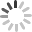Loading...

When you download reports, to make them more readable we report the time taken by a user to complete the quiz in a readable format such as "10 min 30 sec". If you need to translate this into just seconds for doing certain analysis, here is the Excel formula that will translate the “nn hrs nn min nn sec” into a numeric field with the number of seconds.

LOOKUP(9.9E+307,86400*RIGHT("00:00:"&SUBSTITUTE(SUBSTITUTE(SUBSTITUTE(SUBSTITUTE(F3," ",""),"hrs",":"),"min",":"),"sec",""),ROW(INDIRECT("1:"&LEN(F3)))))

Simply download the data we provide to excel and use the above formula to translate time taken to seconds. You would just need to change the two references to F3 to the correct Excel column.

A sample excel sheet contributed by our user John R. is attached.

Attachment (s)

Related Articles: